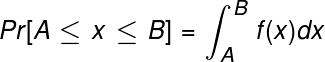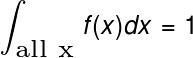# Probability Density Function: Definition, Formula & Examples

Lesson Transcript
Instructor
Aaron Miller

Aaron teaches physics and holds a doctorate in physics.

Expert Contributor
Shawna Smith

Shawna has a master's degree in Education and has taught high school math for over 15 years.

A probability density function is a tool for building mathematical models of real-world random processes. In this lesson, we'll start by discussing why probability density functions are needed in probability theory, then we'll provide its definition and several examples of common probability density functions. Updated: 05/30/2020

## Background on Probability Models

Probability models, which quantify the chances of a random event occurring, are common in everyday life. Take, for example, flipping a fair coin. We believe that if a fair coin is flipped, it has equal chances of landing on heads or on tails. What this means in practice is that if we flip the coin a large number of times, half of the time the coin will show heads and the other half the coin will show tails. Therefore, we assign probability ½ to the outcome heads and ½ to the outcome tails. This is an example of a probability model.

However, assignment of probabilities to certain events does not always work. An example is a game of darts. What is the difference between flipping coins and darts? In particular, a game of darts is a situation where the outcome (the final position of the dart) can take on a continuous range of values.

More specifically, suppose you are throwing darts at a dartboard, and you want to determine the probability of hitting a point that is exactly on the vertical axis, two inches above the center. Like in the coin-flipping example we went over at the outset, the probability we would assign to this outcome is the fraction of darts that hit this precise point after throwing a large number of darts.

However, it's unlikely that any dart hits that exact point, and so even in the limit of an infinite number of throws, the probability of hitting a single point is vanishingly small. Although this issue seems to complicate building a probability model, it isn't consequential to the game of darts in practice because determining the score depends on finding the dart within certain finite regions of the board. So, how can we relate the nearly zero probability of hitting any particular point to the probability of a dart hitting a certain scoring region of the board? A probability density function addresses this problem.

An error occurred trying to load this video.

Try refreshing the page, or contact customer support.

Coming up next: Ratios & Rates: Definitions & Examples

### You're on a roll. Keep up the good work!

Replay
Your next lesson will play in 10 seconds
• 0:03 Background on…
• 1:53 Probability Density Functions
• 3:57 Important General Properties
• 4:58 Some Examples
• 6:45 Lesson Summary
Save Save

Want to watch this again later?

Timeline
Autoplay
Autoplay
Speed Speed

## Probability Density Functions

In order to make a probability model for a scenario where outcomes of random events are numerically valued on a continuous range, like in the dartboard example, a probability density function f(x) must be given. This function provides a way to assign probabilities not to single outcomes, but to ranges of outcomes -- like scoring regions of a dartboard. Scientists and engineers often make an educated guess about the exact form of this function. The independent variable x of this, the probability density function, takes on values within the continuous range of possible outcomes of a random process. Consequently, x is called a continuous random variable. The location of a dart thrown at a dartboard is a continuous random variable because between any two possible locations, you can find another possible outcome.

If we had a probability density function f(x) for darts hitting a dartboard, or any other random process involving a continuous random variable, how would we calculate a probability for an event, such as hitting the bullseye on a dartboard? According to probability theory, the probability of measuring an outcome within a finite range can be calculated by integrating the probability density function over the interval of interest:Pr[A≤ x ≤B] stands for the probability of an outcome within the interval from A to B. For example, foot size in centimeters is a continuous random variable. If you want to know the probability of picking someone with a foot length between 21 and 22 centimeters from a random sample, and you believe the probability density function describing the distribution of foot lengths in centimeters is f(x), then you would integrate f(x) from x = 21 cm to x = 22 cm.

## Important General Properties

This prescription for obtaining probabilities from density functions implies two important properties that a probability density function must have in order to model an actual outcome of a random process. First, a probability density function must be non-negative (i.e., f(x) > 0 for all values x). Second, a probability density function that must obey a rule is called the normalization condition. The normalization condition says:To unlock this lesson you must be a Study.com Member.

## Probability Density Function Defined

A discrete probability model will have countable outcomes. For example: a coin toss has exactly two outcomes. What are some other probability models with countable outcomes?

A continuous probability model will have infinitely many possible outcomes within a given range. You cannot count the precise locations where a dart might land, but you can limit it to a particular area. What are some other probability models with infinitely many outcomes within a given range? What would the range of possible outcomes be? (In other words, what would A and B be for your example?)

Some discrete probability models include:

• rolling a die or several dice
• finding the probability that a person's birthday falls on a particular date
• counting the number of cars that go through an intersection in a given amount of time

Some continuous probability models include:

• rolling a marble and measure the distance it travels (where the range would be from 0 to the farthest possible distance that it could roll)
• the amount of rainfall in a year (where the range would be from 0 to the maximum rainfall possible in a year)

## Probability Density Function Properties

• Why must a probability function be non-negative?
• Why must a probability function obey the normalization condition?

• When calculating probability, the possible probabilities are always between 0% and 100% (between 0 and 1). Therefore, no probability may be negative.
• In any experiment, the sum of the probability of all possible different outcomes must add up to 100% (or, expressed as a decimal, the sum of the outcomes must add up to 1). If we integrate a probability density function over the range of all possible outcomes, we are effectively calculating the sum of all of the probabilities, which must equal 1.

### Register to view this lesson

Are you a student or a teacher?